Question

# Explain Newton's second Law and solve related problems Given a mass of 2.00 kg. Let g...

1. Explain Newton's second Law and solve related problems

Given a mass of 2.00 kg. Let g = the acceleration due to gravity on Earth in SI units. Which of these expressions will yield the weight of the mass on Earth in SI units?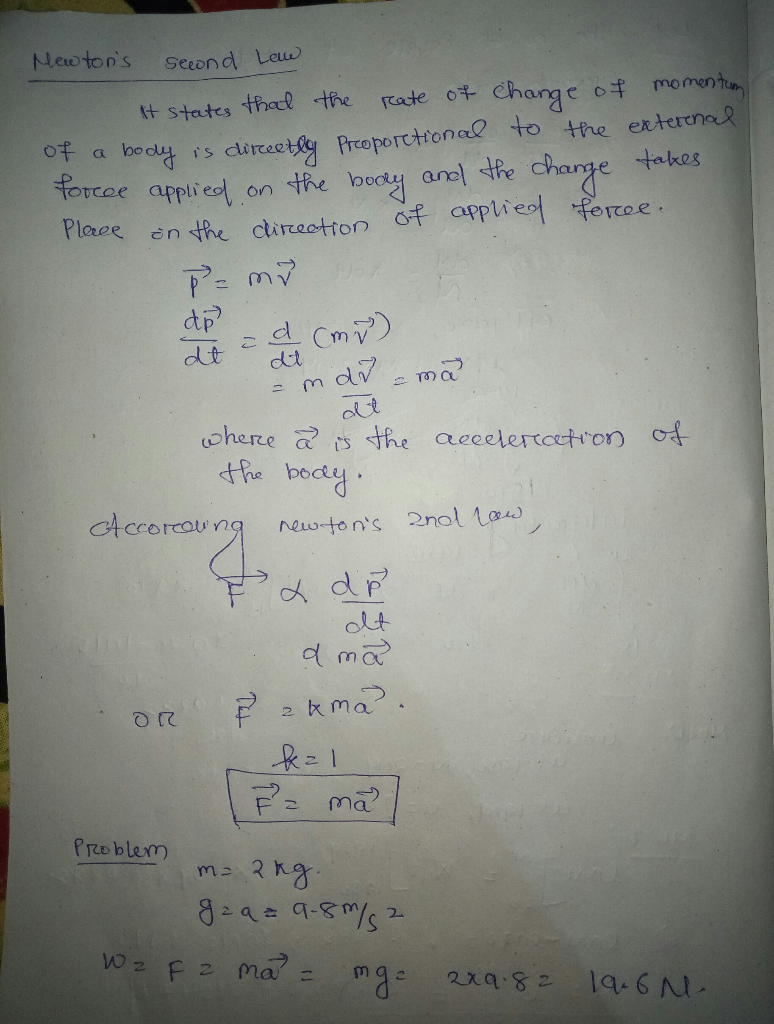#### Earn Coins

Coins can be redeemed for fabulous gifts.

Similar Homework Help Questions
• ### Newton's Second Law: Cart and hanging mass Here is another example of Newton's Second Law. A...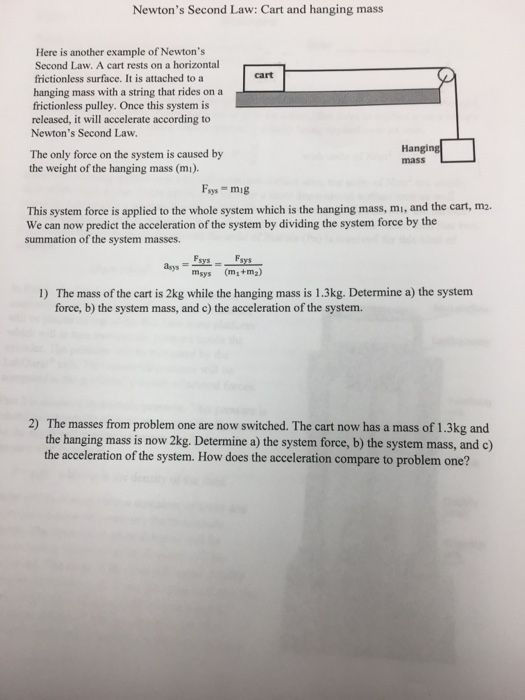Newton's Second Law: Cart and hanging mass Here is another example of Newton's Second Law. A cart rests on a horizontal frictionless surface. It is attached to a cart hanging mass with a string that rides on a frictionless pulley. Once this system is released, it will accelerate according to Newton's Second Law. The only force on the system is caused by Hanging mass the weight of the hanging mass (mi). Fsys mig This system force is applied to the...

• ### Newton's universal law of gravity tells us that F=G 2 Newton's second law tells us that...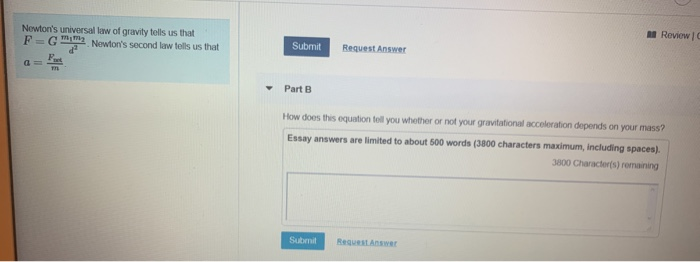Newton's universal law of gravity tells us that F=G 2 Newton's second law tells us that Review Submit Request Answer a = Part B How does this equation tell you whether or not your gravitational acceleration depends on your mass? Essay answers are limited to about 500 words (3800 characters maximum, including spaces 3800 Character(s) remaining Submit Request Answer

• ### In lab, you used an Atwood's Machine to verify Newton's Second Law of Motion. The acceleration...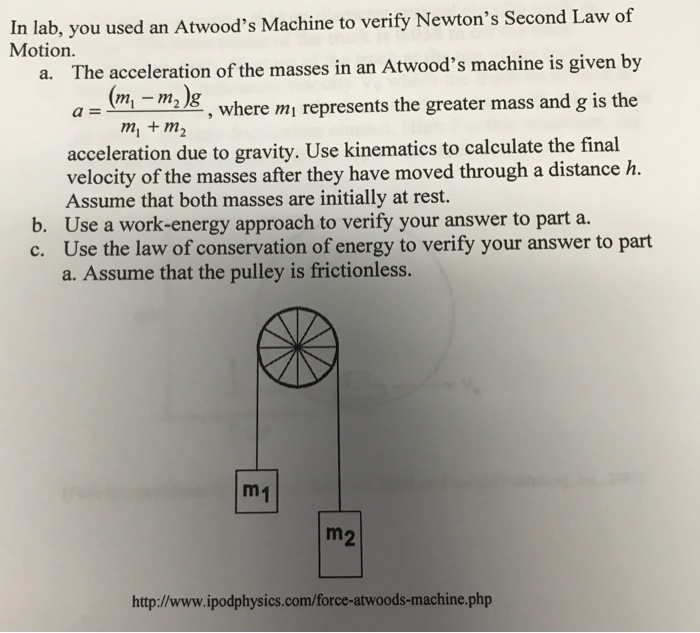In lab, you used an Atwood's Machine to verify Newton's Second Law of Motion. The acceleration of the masses in an Atwood's machine is given by a = (m_1 - m_2)g/m_1 +m_2, where m_1 represents the greater mass and g is the acceleration due to gravity. Use kinematics to calculate the final velocity of the masses after they have moved through a distance h. Assume that both masses are initially at rest. Use a work-energy approach to verify your answer...

• ### 4. Using the Newton's law of gravitation and weight information, Compute the mass of the Earth...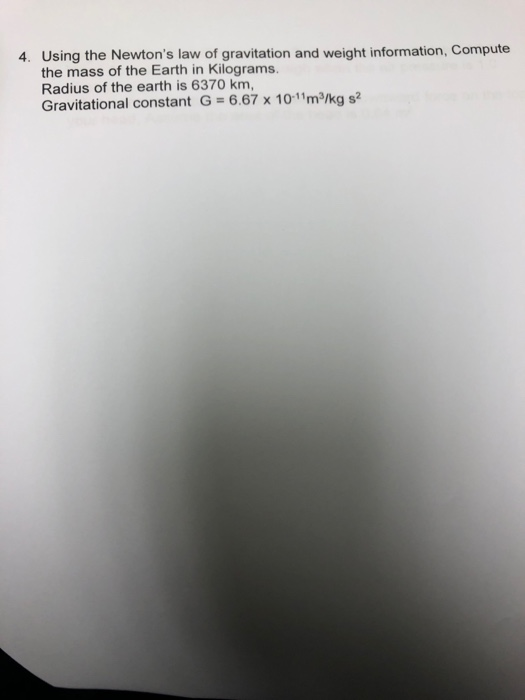4. Using the Newton's law of gravitation and weight information, Compute the mass of the Earth in Kilograms. Radius of the earth is 6370 km, Gravitational constant G = 6.67 x 10-11 m/kg s2

• ### can someone solve c) Problem 3: Newton's third law An apple of mass 0.1 kg falls...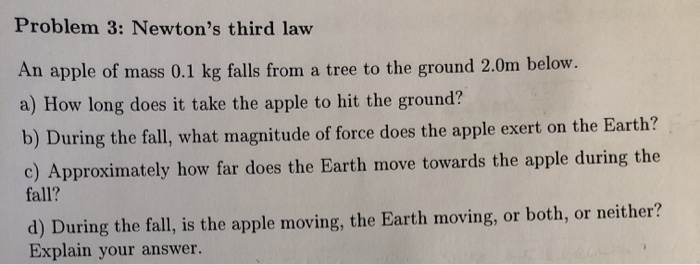can someone solve c) Problem 3: Newton's third law An apple of mass 0.1 kg falls from a tree to the ground 2.0m below. a) How long does it take the apple to hit the ground? b) During the fall, what magnitude of force does the apple exert on the Earth? c) Approximately how far does the Earth move towards the apple during the fall? d) During the fall, is the apple moving, the Earth moving, or both, or neither?...

• ### Solve Part D please Learning Goal: To understand how the conservation of energy and Newton's second...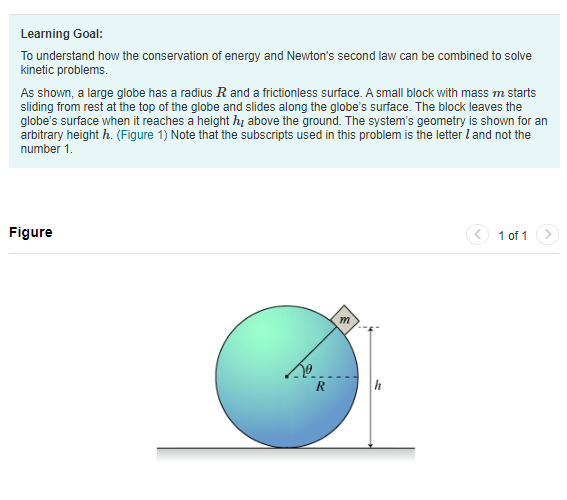Solve Part D please Learning Goal: To understand how the conservation of energy and Newton's second law can be combined to solve kinetic problems. As shown, a large globe has a radius R and a frictionless surface. A small block with mass m starts sliding from rest at the top of the globe and slides along the globe's surface. The block leaves the globe's surface when it reaches a height h above the ground. The system's geometry is shown for...

• ### Concept: Newton's Second Law 2. Consider the spring-pendulum to the right. The spring force is, given...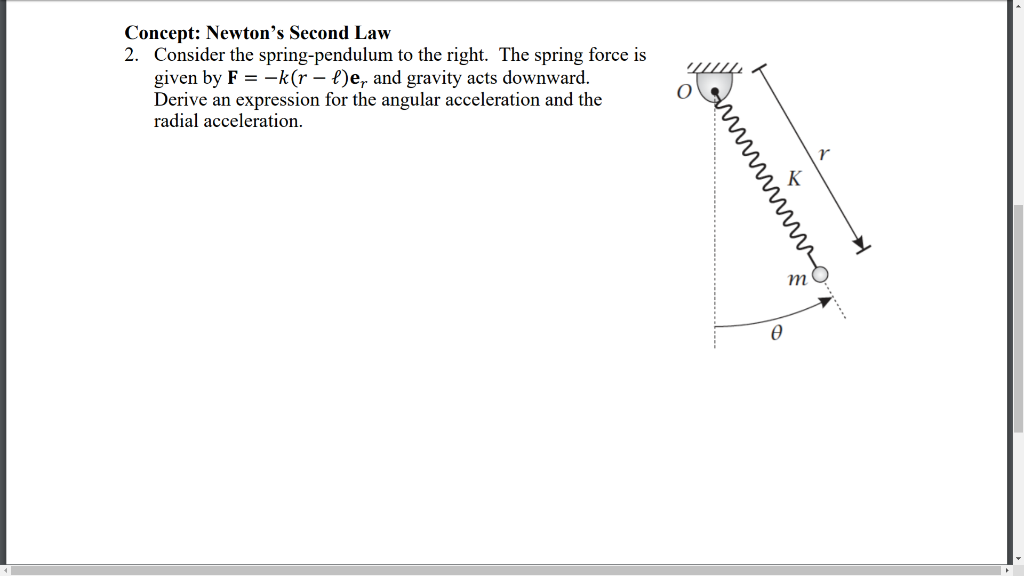Concept: Newton's Second Law 2. Consider the spring-pendulum to the right. The spring force is, given by F--k(r- )e, and gravity acts downward. Derive an expression for the angular acceleration and the radial acceleration nm

• ### 1-9 A rocket has a mass 250010) slugs on earth. Specify a) its mass in SI...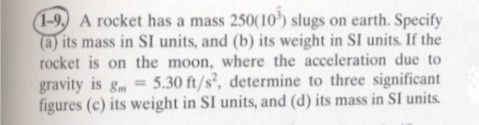1-9 A rocket has a mass 250010) slugs on earth. Specify a) its mass in SI units, and (b) its weight in SI units. If the rocket is on the moon, where the acceleration due to gravity is g-5.30 ft/s, determine to three significant figures (c) its weight in SI units, and (d) its mass in SI units

• ### Lab Activity: Newton's Second Law The goal of this exercise is to examine a system of...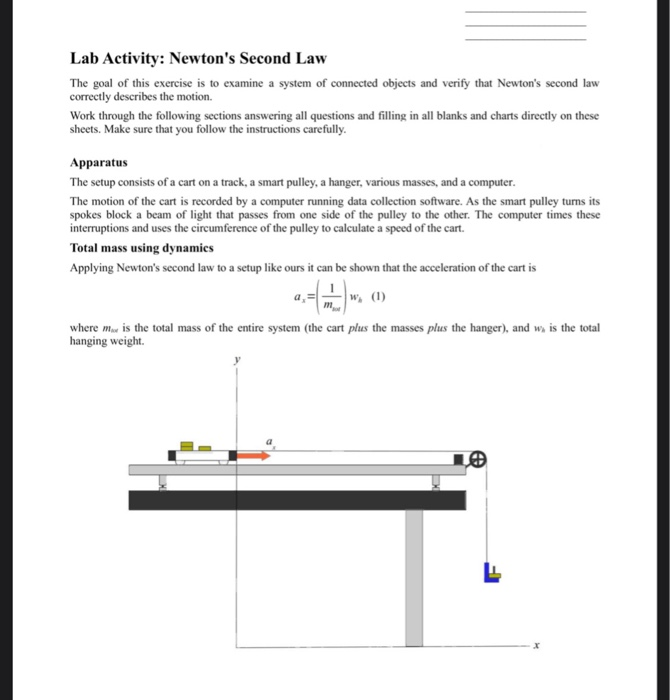Lab Activity: Newton's Second Law The goal of this exercise is to examine a system of connected objects and verify that Newton's second law correctly describes the motion. Work through the following sections answering all questions and filling in all blanks and charts directly on these sheets. Make sure that you follow the instructions carefully. Apparatus The setup consists of a cart on a track, a smart pulley, a hanger, various masses, and a computer. The motion of the cart...

• ### 2. According to Newton's Law of Universal Gravitation, the gravitational force on an object of ma...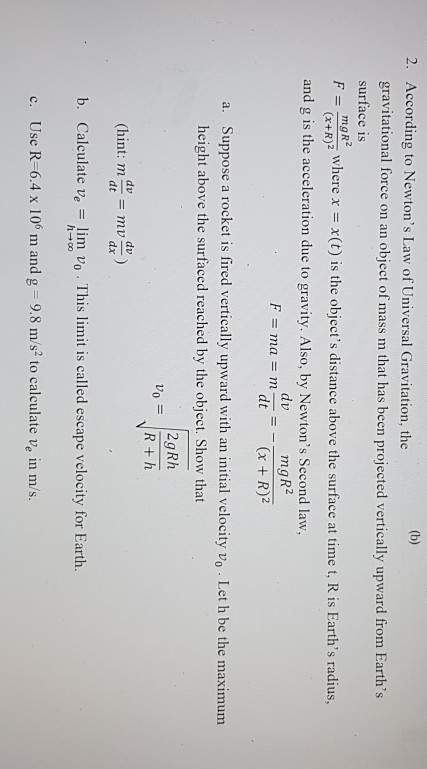Can you please give me the whole solution for this question! Thanks 2. According to Newton's Law of Universal Gravitation, the gravitational force on an object of mass m that has been projected vertically upward from Earth's surface is F( is the objer s distan boe he urfac at time t, Ris Earth's radius, ngR (x+R)2 and g is the acceleration due to gravity. Also, by Newton's Second law, mgR2 (x +R)2 dv F = mal = m dt =...